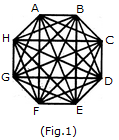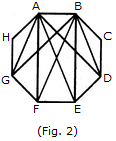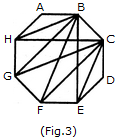# Non Verbal Reasoning - Analytical Reasoning - Discussion

Discussion Forum : Analytical Reasoning - Section 1 (Q.No. 27)
27.

What is the number of triangles that can be formed whose vertices are the vertices of an octagon but have only one side common with that of octagon?

64
32
24
16
Explanation:When the triangles are drawn in an octagon with vertices same as those of the octagon and having one side common to that of the octagon, the figure will appear as shown in (Fig. 1).Now, we shall first consider the triangles having only one side AB common with octagon ABCDEFGH and having vertices common with the octagon (See Fig. 2).Such triangles are ABD, ABE, ABF and ABG i.e. 4 in number.Similarly, the triangles having only one side BC common with the octagon and also having vertices common with the octagon are BCE, BCF, BCG and BCH (as shown in Fig. 3). i.e. There are 4 such triangles.

This way, we have 4 triangles for each side of the octagon. Thus, there are 8 x 4 = 32 such triangles.

Discussion:
5 comments Page 1 of 1.

Pri said:   5 years ago
I can see total 8 triangles with one side of octagon AB. Line AD and BG are meeting or crossing each other at one point which is also making one more triangle. In same way other lines are also making three more triangles with AB side. So total 8 triangles on AB. Please let me know whether Im wrong or right.

Sudarshan said:   5 years ago
Because ABH become 2 sides common.

Arpan said:   7 years ago
What about ABH? why not considering it taking AB common?

Arpan said:   7 years ago
What about triangle ABH (taking AB as common) ?

Ragav said:   10 years ago
In figure 2, A triangle ABX with one side common with octagon (ie. AB) can be formed such that, X is the midpoint (intersection of triangles ABG & ABD. Why it is not considered?